Beyond Minkowski ansatz

There are two examples of correct polynomials that don’t fit into ansatz stated below.
I think I have shown these examples to Alessio in April.

More info in the notes of my talk (page 2, polynomials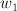$w_1$ and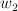$w_2$): pdf (or follow the link from here).

Both examples are degenerations of projective space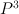$P^3$ (grdb).

================================================================================
Period sequence 12
First 10 period coefficients: [1, 0, 0, 0, 24, 0, 0, 0, 2520, 0]
The PF operator has N=3, r=4
This sequence has a smooth toric Fano representative
It arises from the following polytopes [(PALP id, grdb id, smoothness)]:
(0, 547386, ‘smooth’)
The PF operator for this sequence is:
256*t^4*D^3 + 1536*t^4*D^2 + 2816*t^4*D + 1536*t^4 – D^3
================================================================================

So we start from the familiar Laurent polynomial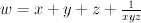$w = x + y + z + \frac{1}{xyz}$

I. Argument against “lattice” decomposition.

a. make monomial transformation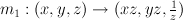$m_1: (x,y,z) \to (xz,yz,\frac{1}{z})$

w goes to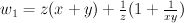$w_1 = z (x+y) + \frac{1}{z} (1+ \frac{1}{xy})$

b. mutate by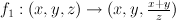$f_1: (x,y,z) \to (x,y,\frac{x+y}{z})$$w_1$ goes to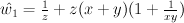$\hat{w_1} = \frac{1}{z} + z (x+y) (1 + \frac{1}{xy})$

Since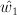$\hat{w_1}$ is derived from w by transformation from group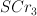$SCr_3$ it is a mirror for projective space$P^3$ (“weak Landau-Ginzburg model” in Przyalkowski’s notations).

Newton polygon of$\hat{w_1}$ is fan polytope of a Gorenstein toric variety – (grdb).
This variety is anticanonical cone over smooth quadric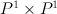$P^1 \times P^1$, i.e. a cone over section of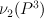$\nu_2(P^3)$ ($P^3$ embedded into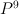$P^9$ by complete linear system of quadrics),
and hence it is a geometric degeneration of$P^3$.

Consider the quadrangular face, corresponding to a singular point. Restriction of$\hat{w_1}$ to this face is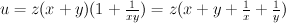$u = z (x+y) (1 + \frac{1}{xy}) = z ( x + y + \frac{1}{x} + \frac{1}{y} )$. It is not friendly to Minkowski ansatz’s condition of lattice Minkowski decomposition (this is exactly the example of Minkowski decomposition that is not a lattice Minkowski decomposition given in the definition of the ansatz).

II. Argument against “admissible triangles” and decomposing polytopes completely.

Example$w_2$ from the same notes.

This one is degeneraiton to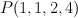$P(1,1,2,4)$ (grdb).

By monomial transformation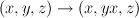$(x,y,z) \to (x,yx,z)$

transform w to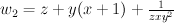$w_2 = z + y (x+1) +\frac{1}{z x y^2}$

then by mutation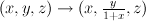$(x,y,z) \to (x, \frac{y}{1+x}, z)$

transform$w_2$ to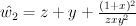$\hat{w_2} = z + y + \frac{(1+x)^2}{z x y^2}$$P(1,1,2,4)$ is embedded as a quadric in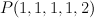$P(1,1,1,1,2)$ by linear system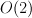$O(2)$, so it is a degeneration of a general quadric in this space i.e.$P^3$.
This variety$P(1,1,2,4)$ is also the anticanonical cone over singular quadratic surface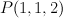$P(1,1,2)$.

Restriction to the face equivalent to this surface is equal to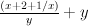$\frac{(x+2+1/x)}{y} + y$, so it is not admissible.

Z. How to tune the ansatz?

Universal fix:
allow change of the lattice after creating some of the good polynomials

less universal:
a. Allow non-lattice Minkowski decompositions
AND/OR
b. Increase the set of admissible figures

Update on June 22:
III. Examples further beyond

By combining technique from examples in this and previous post we can construct some more sophisticated
mirrors for Tom and Jerry. These mirrors should correspond to degenerations of these guys to Gorenstein cones over singular (Gorenstein or not) del Pezzo surfaces of degree 6.
I’ll write only numerical details and maybe will provide some geometry later in the comment.

Start from a honeycomb and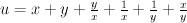$u = x+y+\frac{y}{x}+\frac{1}{x}+\frac{1}{y} +\frac{x}{y}$

Using cluster transformations it can be transformed to mirrors constructed from Gorenstein toric degenerations of del Pezzo surface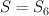$S = S_6$.

first to pentagon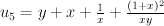$u_5 = y + x + \frac{1}{x} + \frac{(1+x)^2}{xy}$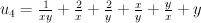$u_4 = \frac{1}{xy} + \frac{2}{x} + \frac{2}{y} + \frac{x}{y} + \frac{y}{x} + y$

then to triangle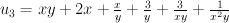$u_3 = xy + 2x + \frac{x}{y} + \frac{3}{y} + \frac{3}{xy} + \frac{1}{x^2y}$

The triangle is fan polytope of Gorenstein weighted projective plane P(1,2,3).

We can mutate it further to get non-Gorenstein weighted projective plane P(1,3,8)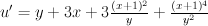$u' = y + 3x + 3\frac{(x+1)^2}{y} +\frac{(x+1)^4}{y^2}$

Then we choose G equal to 2 or 3
and take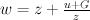$w = z + \frac{u+G}{z}$

This will be weak mirror for Jerry or Tom,
all underlying toric threefolds are Gorenstein.

Last two are P(1,2,3,6) (grdb) and P(1,3,8,12) (grdb).

Triangles may be Minkowski decomposable only when they are multiples of smaller triangles, which is not the case in these examples.

Altmann’s results on relations between Minkowski decompositions and deformations does not apply here since we have non-isolated singularity (it is a cone over already singular space).